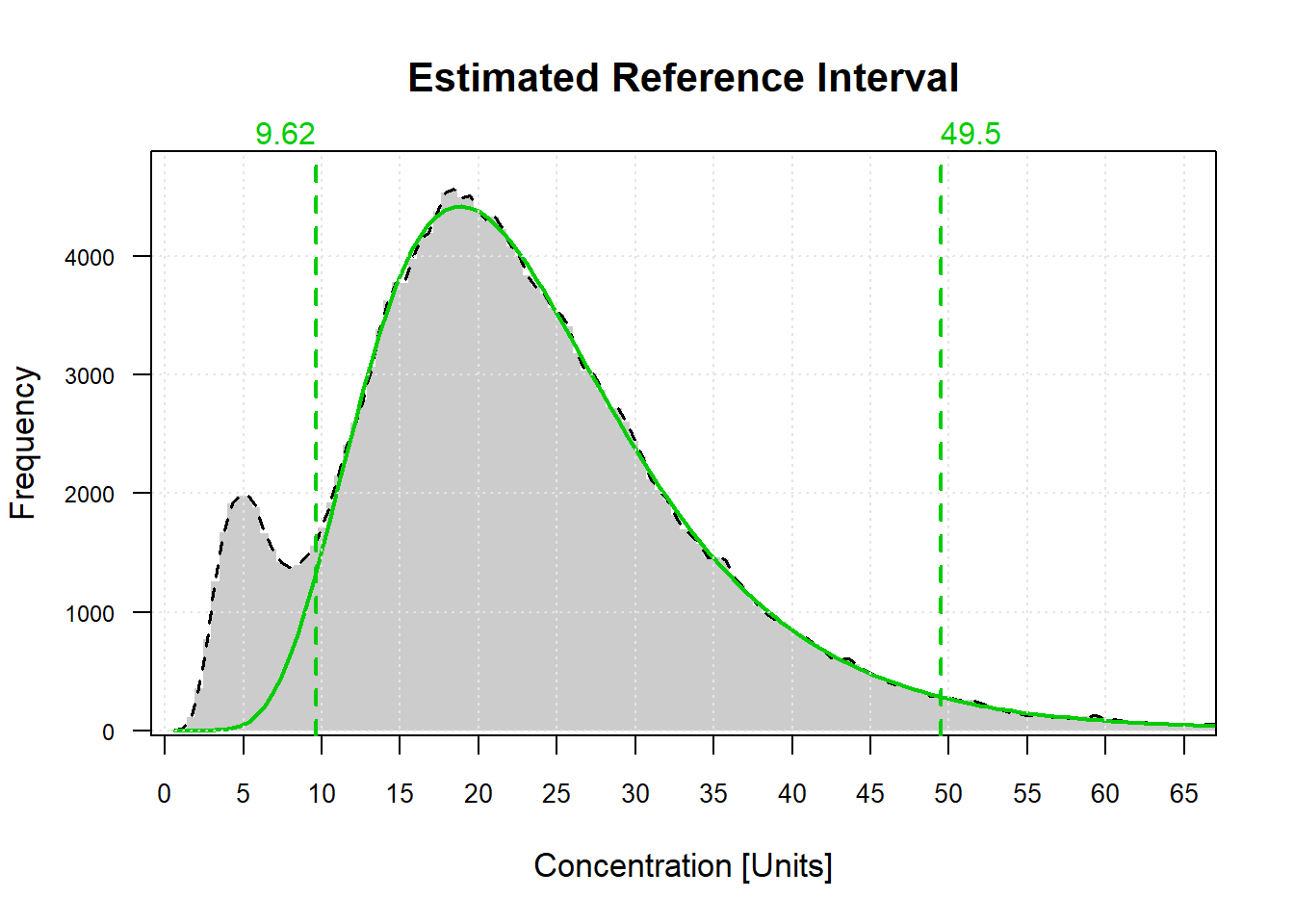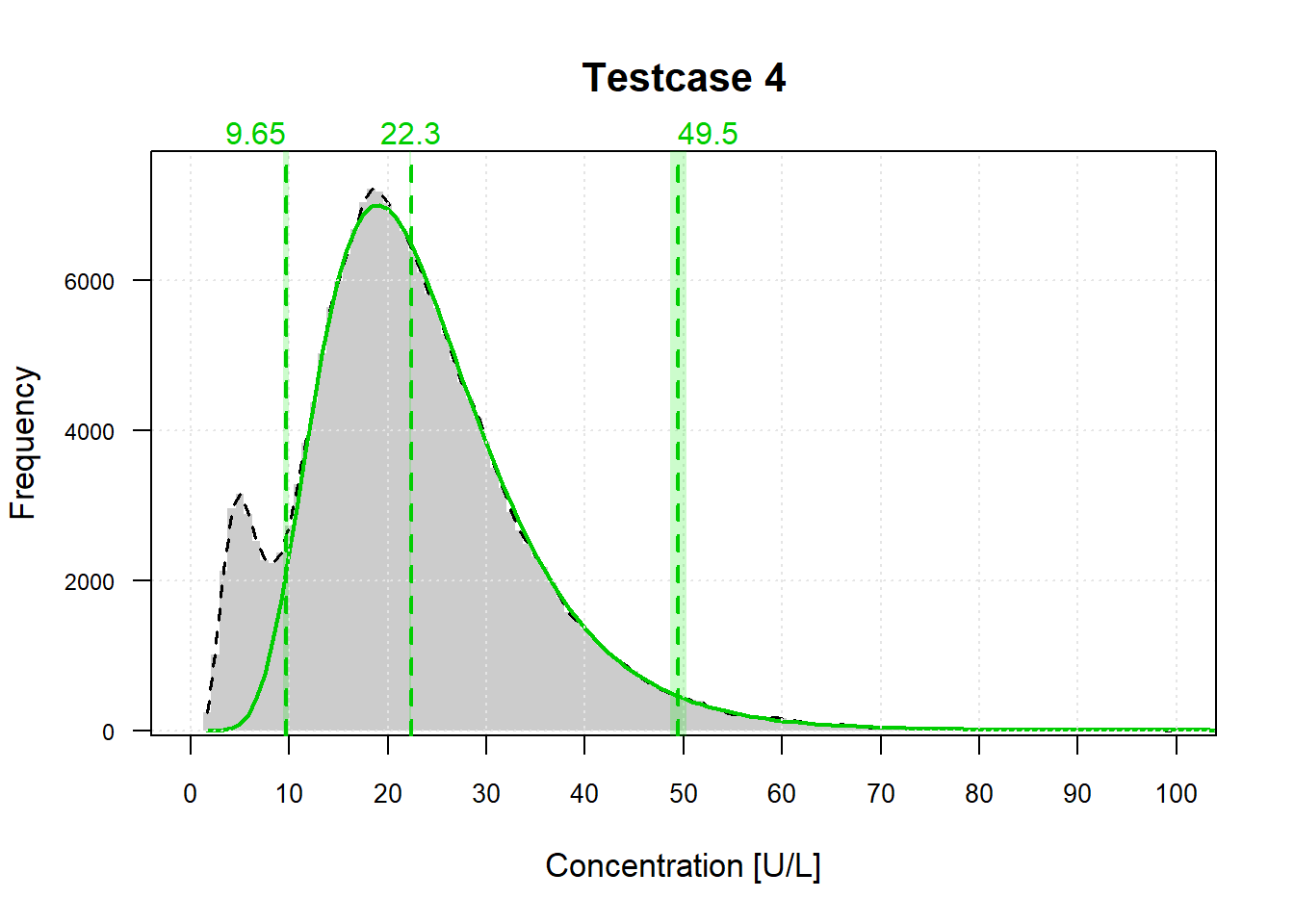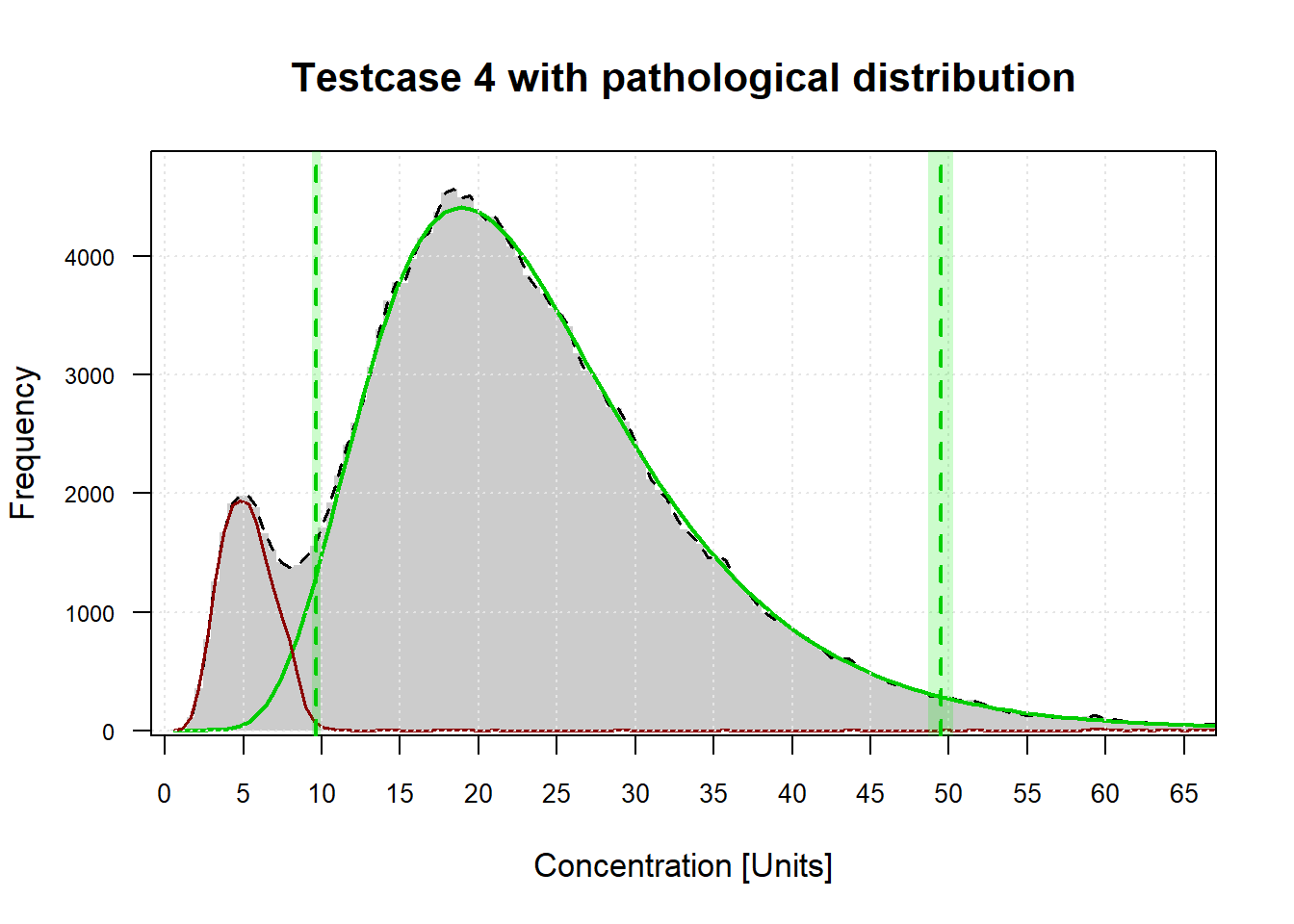## Introduction

The R-package refineR implements the recently published, state-of-the-art indirect method, refineR (Ammer et al., 2021, description of refineR v1.0) which was developed for the estimation of reference intervals using Real-World Data (RWD) [https://doi.org/10.1038/s41598-021-95301-2]. It takes routine measurements of diagnostic tests, containing pathological and non-pathological samples as input and uses sophisticated statistical methods to derive a model describing the distribution of the non-pathological samples. This distribution can then be used to derive reference intervals. The R-package offers various convenience functions to estimate the model, compute reference intervals, as well as to print and plot the estimated results.

## Input Data

The R-package refineR comes with five simulated datasets that mimick routine measurements of biomarkers observed in laboratory practice. These datasets describe a mixed distribution containing non-pathological and pathological values. The datasets differ in number of samples, pathological fraction and underlying non-pathological distribution. A detailed description of the different testcases can be found in the package documentation.

Prefiltering, cleaning, and possible partitioning of the extracted data from the laboratory information system is not included in the refineR package and has to be carried out in advance. The simulated datasets used here for showcasing the application reflect already preprocessed datasets.

For demonstrating the application of the functions provided by the refineR package, testcase 4 is used as an example dataset:

# load refineR package and load data
library(refineR)
head(testcase4)
##  17.76419 20.34425 42.40541 23.01746 23.57971 45.21698

## Model Estimation and Presentation of Results

### Default Settings

The main function of refineR is findRI() that takes an input data set and estimates the model parameters lambda, mu and sigma of a Box-Cox transformed normal distribution that best explains the non-pathological distribution. The optimization is carried out via a multi-level grid search to minimize the cost function (negative log-likelihood with regularization). For a detailed description of the method (v 1.0), please refer to Ammer et al., 2021. The findRI() function takes the following main function arguments:

• Data … (numeric) values specifying data points comprising pathological and non-pathological values
• model … (character) specifying the applied model (can be either BoxCox (default, 1-parameter Box-Cox transformation), modBoxCoxFast or modBoxCox (2-parameter Box-Cox transformation), modBoxCoxFast offers faster but less accurate results than modBoxCox
• NBootstrap … (integer) specifying the number of bootstrap repetitions
• seed … (integer) specifying the seed used for bootstrapping

To run the model estimation using the default parameters just the (pre-processed) input data is required (Data):

# run refineR estimation and print resulting RWDRI object
fit <- findRI(Data = testcase4)
print(fit)
##
## Reference Intervals
## ------------------------------------------------
## lower limit [ 2.5% perc]: 9.62
## upper limit [97.5% perc]: 49.5
##
## Model Parameters
## ------------------------------------------------
##      method: refineR (v1.6.0)
##       model: BoxCox
##      N data: 100000
##     rounded: no
##   point est: fullDataEst
##      lambda: 0.0553
##          mu: 3.38
##       sigma: 0.496
##       shift: 0
##        cost: -36.6
## NP fraction: 0.909

The print() method comprises an overview of the model estimation results. First, it shows the estimated lower and upper reference limit, per default the 2.5% and 97.5% percentiles. Second, it depicts information about the used refineR version, the applied model, as well as the number of of data points (N data) and whether the input data was rounded or not (rounded). Further, it shows the estimated model parameters (lambda, mu, sigma, shift), the estimated costs and the estimated fraction of non-pathological values (NP fraction).

To calculate the estimated reference intervals, the function getRI() can be used. Per default, again the 2.5% and 97.5% percentiles are computed using the estimated model parameters.

# compute reference intervals using the estimated model parameters
getRI(fit)
##   Percentile PointEst CILow CIHigh
## 1      0.025  9.61956    NA     NA
## 2      0.975 49.50094    NA     NA

We can now also take a look at the result by using the plot() function.

# plot the estimated model
plot(fit)The plot shows the raw input data (gray histogram) as well as the estimated model for the non-pathological distribution (green curve) and the estimated reference intervals (per default again the 2.5% and 97.5% percentiles) as green dashed lines.

#### Computation of Confidence Intervals

As mentioned, the findRI() function can take additional function arguments. To calculate confidence intervals for the estimation, bootstrapping is required. The number of bootstrap iterations can be set by the argument NBootstrap (default: 0). For demonstration purposes and due to the increased computation time, we used a small number of iterations (NBootstrap = 20). However, for use in real-world analysis, we recommend to use NBootstrap >= 200. When using bootstrapping, the print() function then also gives the estimated confidence intervals for the reference limits (with a default confidence level of 95%).

# run refineR estimation with 20 bootstrap iterations
fit.bs <- findRI(Data = testcase4, NBootstrap = 20)
print(fit.bs)
##
## Reference Intervals
## ------------------------------------------------
## lower limit [ 2.5% perc]: 9.62 (9.39; 9.92)
## upper limit [97.5% perc]: 49.5 (48.7; 50.3)
##
## Model Parameters
## ------------------------------------------------
##      method: refineR (v1.6.0)
##       model: BoxCox
##      N data: 100000
## N bootstrap: 20
##     rounded: no
##   point est: fullDataEst
##      lambda: 0.0553
##          mu: 3.38
##       sigma: 0.496
##       shift: 0
##        cost: -36.6
## NP fraction: 0.909

#### Estimation using Two-Parameter Box-Cox Transformation

In addition to the one-parameter Box-Cox transformation, the refineR algorithm also offers the option to use the two-parameter (modified) Box-Cox transformation. This model takes an additional shift parameter to better model skewed distributions that are shifted away from zero.

# run refineR estimation with alternative model (two-parameter (modified) Box-Cox transformation)
fit.mbc <- findRI(Data = testcase4, model = "modBoxCox")
print(fit.mbc)
##
## Reference Intervals
## ------------------------------------------------
## lower limit [ 2.5% perc]: 9.84
## upper limit [97.5% perc]: 48.9
##
## Model Parameters
## ------------------------------------------------
##      method: refineR (v1.6.0)
##       model: modBoxCox
##      N data: 100000
##     rounded: no
##   point est: fullDataEst
##      lambda: 0.204
##          mu: 4.03
##       sigma: 0.892
##       shift: 3.32
##        cost: -37
## NP fraction: 0.905

#### Plot Function Arguments

The plot() function can take additional function arguments as well:

• x … (object) of class RWDRI, estimated model
• RIperc … (numeric) value specifying the percentiles, which define the reference interval
• Nhist … (integer) number of bins in the histogram (derived automatically if not set)
• showCI … (logical) specifying if the confidence intervals are shown
• showPathol … (logical) specifying if the estimated pathological distribution shall be shown
• showBSModels … (logical) specifying if the estimated bootstrapping models shall be shown
• showValue … (logical) specifying if the exact value of the estimated reference intervals shall be shown above the plot
• CIprop … (numeric) value specifying the central region for estimation of confidence intervals
• pointEst … (character) specifying the point estimate determination: (1) using the full dataset (fullDataEst), (2) calculating the median from all bootstrap samples (medianBS),(3) calculating the mean from all bootstrap samples (“meanBS”), option (2) and (3) only work if NBootstrap>0
• scalePathol … (logical) specifying if the estimated pathological distribution shall be weighted with the ratio of pathol/non-pathol
• xlim … (numeric) vector specifying the limits in x-direction
• ylim … (numeric) vector specifying the limits in y-direction
• xlab … (character) specifying the x-axis label
• ylab … (character) specifying the y-axis label
• title … (character) specifying plot title

When plotting a model estimated with bootstrapping, the confidence intervals are shown per default as light green region. Further, you can specify if you want to show the estimate for the full data set (fullDataEst), or the median (medianBS) or mean (meanBS) of all bootstrap results by setting the argument pointEst. Additionally, you can adjust for example the x-limit, the x-label and the title:

# plot estimated model with bootstrapping with adjusted function arguments
plot(fit.bs, RIperc = c(0.025, 0.5, 0.975), pointEst = "medianBS", xlim = c(0,100), xlab = "Concentration [U/L]",
title = "Testcase 4")If you also want to show the estimated “pathological distribution”, set the showPathol argument to TRUE. This then adds a red curve to the plot, representing the difference between the raw histogram and the estimated model of the non-pathological distribution, i.e. interpretation should be taken with care.

If you don’t want to show the estimated reference limits, you can disable this, by setting showValue to FALSE.

# plot estimated model with bootstrapping showing the difference between raw input data and estimated model
#       (i.e. 'pathological distribution'), wihtout showing the estimated reference limits
plot(fit.bs, showPathol = TRUE, showValue = FALSE, pointEst = "medianBS",
title = "Testcase 4 with pathological distribution")